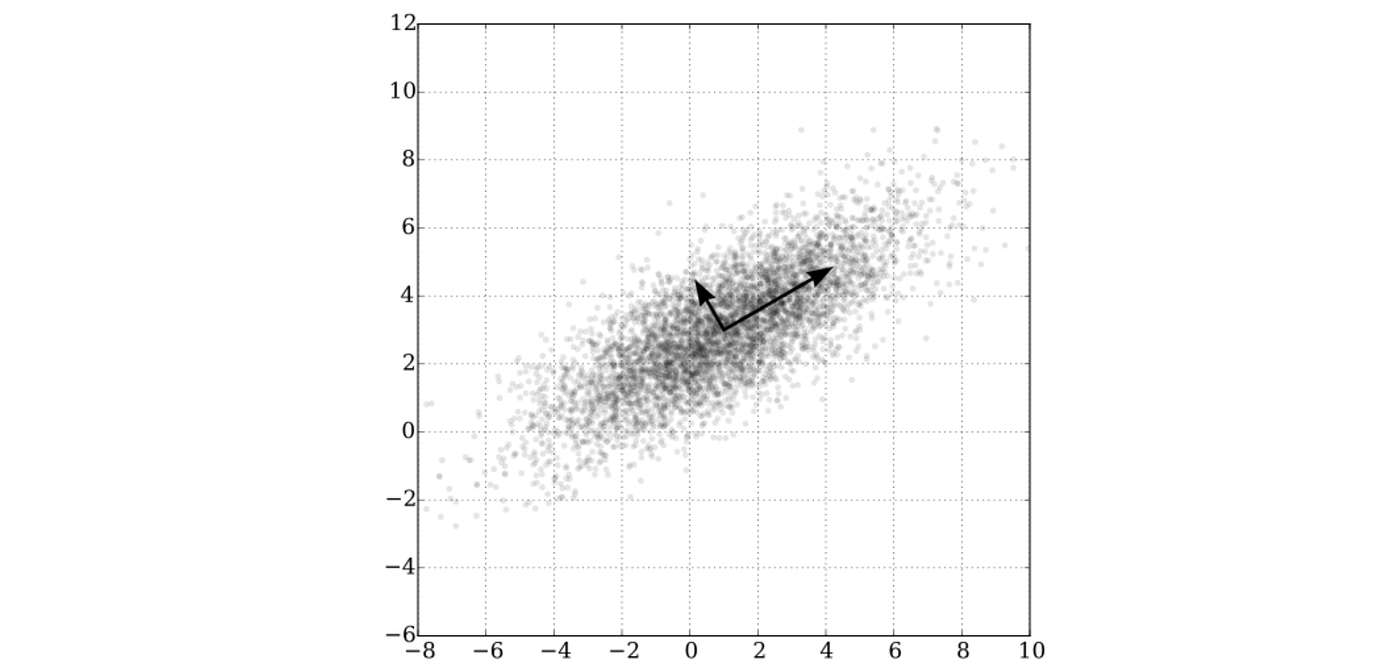PCA over a bivariate Gaussian distribution centered at (1,3). Image by Nicolás Guarín

# The Math of Principal Component Analysis (PCA)

## Using two different strategies rooted in linear algebra to understand the most important formula in dimensionality reduction

This article assumes the reader is comfortable with the contents covered in any introductory linear algebra course — orthogonality, eigendecompositions, spectral theorem, Singular Value Decomposition (SVD)…

Confusion of the proper method to do Principal Component Analysis (PCA) is almost inevitable. Different sources espouse different methods, and any learner quickly deduces that PCA isn’t really a specific algorithm, but a series of steps that may vary, with the final result being the same: data that is simplified into a more concise set of features.

After talking about the basic goal of PCA, I’ll explain the mathematics behind two commonly shown ways to calculate PCA. The first one involves creating a covariance matrix (if that makes you uncomfortable, don’t worry, I’ll explain it) and doing some eigen-calculations on it.

The second involves the Singular Value Decomposition (SVD). Of course, if you’re more comfortable with either one of these intuitions, you can go ahead and just understand it that way, but the big point of this article is to give an overview of how the two ways of seeing it are exactly equivalent.

## The Goal of Principal Component Analysis

It is important to first have a vague sense of what PCA is trying to achieve.

It is trying to reduce the dimensionality of the input data. But what does this mean? Let’s take a specific example. Say we have two roughly correlated features, collected from a mass survey of Canadian citizens: personal happiness and personal achievement.

As we can see, these two features are highly correlated — people with high personal achievement are likely to be happier, and vice versa.

Let’s say that these two features are just a segment of a much larger dataset with many, many more features (x3, x4… xn) associated with the answers to different questions on the survey. Say we have so much data that our data analysis technology is starting to falter, and we want to see ways in which we can reduce the size of our dataset.

Looking over the graphs of all sorts of questions, we see that personal happiness and personal achievement are highly correlated. We could save a lot of space if we could combine these two features into one feature, something like “personal contentedness”.

This is a “dimensionality reduction” problem, perfect for Principal Component Analysis. We want to analyze the data and come up with the principal components — a combined feature of the two.

We can do this by drawing a vector through these data points and projecting each point onto the line we create. We are transferring our data in two dimensions to only needing one dimension.

Now, our data can be shown on a number line, with each of our projected points now only requiring a single coordinate to locate.

This dimensionality reduction will sacrifice some information, but what we are trying to do is find the line that minimizes the loss of information.

We gauge how good a line is at retaining information by using the variance of the points. That is, the average distance each point is from the mean. A good line will have maximized variance — it best preserves the locations of each point. Let’s see a couple of examples.

We can see that with low variance, we erase much of the most important data, and the reduced dataset barely resembles our 2D data.

With high variance, we see that our one-dimensional number line quite effectively retains information, showing a more complete distribution of points.

We are trying to find the vector (line) through our dataset that maximizes the variance. This is a lot harder and is essentially the aim of the two mathematical formulas I will be discussing.

*This all applies in higher dimensions, when we want to reduce some n-dimensional data-space into some k-dimensional dataspace, in which instead of plotting the optimal line, we plot the optimal k-dimensional hyperplane through n-dimensional space.

First, I’ll tackle the PCA algorithm without any concepts of Singular Value Decomposition (SVD) and be looking at it the “eigenvector way”.

## The Eigenvectors of the Covariance Matrix Method

This is perhaps the most common method for computing PCA, so I’ll start with it first. It relies on a few concepts from statistics, namely the covariance matrix. But first, we need to set the format of our input data.

First of all, continuing with our survey example, we have surveyed 100 participants. Each of these participants has a recorded answer for both the ‘happiness’ and ‘achievement’ survey questions.

We represent this as the input matrix X, which is 100 x 2 long. Each row is a new training example (individual) and each column is that individual’s happiness (x1) and achievement (x2).

## The Covariance Matrix

What we want to do is construct a 2 x 2 covariance matrix which represents the covariance between each variable. I’ll explain exactly why we are searching for the covariance matrix shortly. But first, let’s explore. In pseudo-mathematics, a covariance matrix looks something like this:

The covariance measures the variance between different variables. A negative covariance between some variables a and b means that when a goes up, b goes down. A positive covariance means that when a goes up, so does b.

In our scenario, the top left will measure the covariance between happiness and happiness — when we are measuring the covariance between the same things, we are just measuring variance.

And our off-diagonal covariances are the same — the covariance between a and b is the same as the covariance between b and a (order doesn’t matter).

So our desired covariance matrix simplifies to:

Our covariance matrix, and all covariance matrices, are symmetric, with variances along the diagonal. Covariance can be seen as diagonal variance if that helps anything. Covariance gives detail on the orientation of data, and variance gives detail on the average distance from the mean.

## Constructing the Covariance Matrix

We can simply and directly construct the covariance matrix by doing a simple matrix operation on our input matrix X.

Keeping in mind the exact formula for calculating the covariance of two features (each with N examples):

Using this, we can come up with a much more streamlined matrix multiplication to calculate covariance. Notice that our formula for covariance sums over the products of the differences of each feature and its corresponding mean.

What we can do to ‘automate’ the process of subtracting the mean from each feature. We can normalize our matrix by subtracting the mean of each feature from each training example.

This leaves us with a modified, “normalized” X, where the mean over all the columns (examples) is 0. In datasets with features that range in values (ages, days of the week, money in a savings account) we might also want to divide each column by the standard deviation of the column, but since both of our data is 1–10 we don’t have to do that.

Now, to calculate our covariance matrix, we can simply multiply the transpose of our normalized matrix Xnorm.

By doing row by column matrix multiplication, we see that we’re exactly calculating our covariance formula for each of the four entries into our 2 x 2 output. Remember that the summation is exactly equal to a dot product, and everything will fall into place.

All that we’re missing is to divide each covariance summation by the amount of examples N, and we can do that by dividing our 2 x 2 output by N. So, the total formula for any covariance matrix (and this works for any amount of dimensions!): is as follows: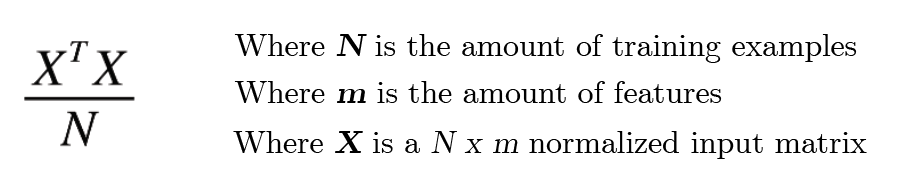You might sometimes see (XX^T)/N, but this is when X is a m x N normalized input matrix (training examples as columns instead of rows). It is like this is Gilbert Strang’s lecture on PCA and Eckart-Young. Both ways work and the outcome is the same — a m x m covariance matrix.

It never ceases to amaze just how much matrix notation can help simplify otherwise convoluted formulas. Imagine having to write out the formula for a convolution matrix using summations!

## Eigenvectors of the Covariance Matrix and Spectral Theorem

This intuition was inspired by user Amoeba on Cross Validated.

I’ll tell you upfront the answer to calculate the principal component (the vector that corresponds to the results for each training example along our new line of personal contentedness).

Our “principal component”, or a vector through 2D space that maximizes the variance of all projected points onto it, is the eigenvector of the covariance matrix associated with the largest eigenvalue.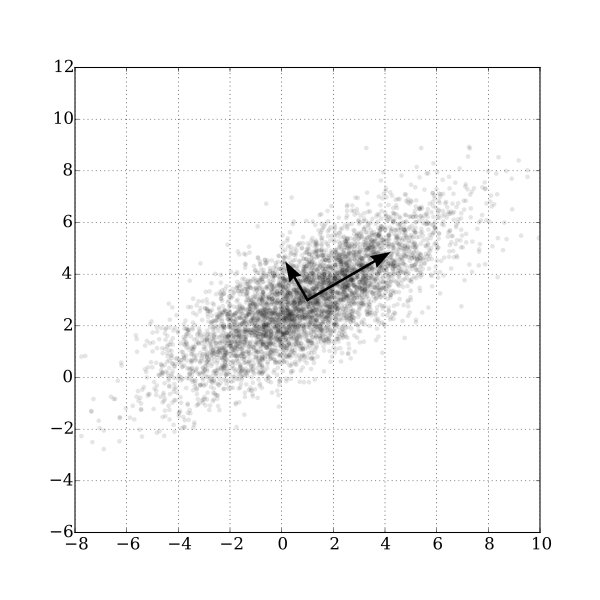To put it simply, our covariance matrix might have two eigenvectors denoted by these two arrows. We take the one with longer length (therefore, a larger eigenvalue, since we treat eigenvectors in PCA as unit length) to be our principal component. But why do our eigenvectors go in this direction in the first place?

The much, much harder question is why this is. There are several ways to look at the intuition for why this works. None of them are extremely easy to understand, and I’ll try to use a method to do with the spectral theorem.

The spectral theorem is covered in the Eigen-things unit in any introductory linear algebra course, so I won’t go over the details here. The summarization is that any symmetric matrix can be factorized into: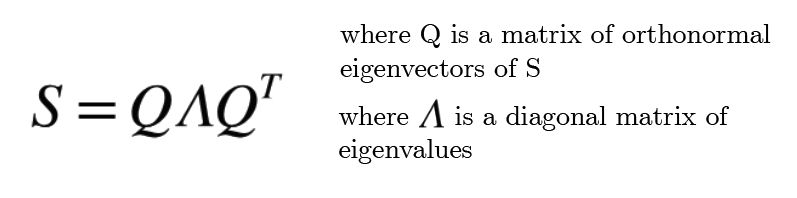It’s a generalization of the more A = QΛQinv factorization since symmetric matrices have orthogonal (and therefore, orthonormal by dividing by the lengths of each vector) eigenvalues. The inverse of an orthonormal matrix is equal to its transpose.

Furthermore, since any symmetric matrix has a full set of n eigenvectors, all of which are orthogonal (and can be easily made orthonormal by dividing by the length of each vector), we can use the eigenvectors as a basis.

Since our covariance matrix C is and always will be symmetric, the spectral theorem applies as well to our covariance matrix. We can come up with an eigenbasis from the columns of Q.

To make this concrete, let’s make a covariance matrix C with concrete values. Let’s continue with our example of the happiness-achievement problem.

We can now compute the eigenvectors and eigenvalues of this covariance matrix C, and see what happens when we set these vectors as our basis.

We calculate our eigenvectors (which are orthogonal by default since we are dealing with symmetric matrices) and convert them to orthonormal by dividing by the length of the vectors. We can calculate the eigenvalues to accompany our new orthonormal vectors by multiplying the eigenvalues by multiplying each by the length of the corresponding vector (dividing by the length and multiplying cancels out).

Now, let’s see if we can switch to an eigenbasis — so, our two eigenvectors become our basis vectors — our (1, 0) = (0.83, 0.55) and (0, 1) = (-0.55, 0.83). We can plot these basis vectors on a graph in the cartesian coordinate space and see that they are indeed orthogonal and unit length — just like our normal unit vectors, but rotated.

But now it’s important to note we’re going to occupy eigenbasis coordinate system where are (1, 0) actually are equivalent to the vectors above in the cartesian system.

So now, assuming the eigenbasis coordinate system, how can we express our matrix C? Well, remembering the critical and definitive rule of eigenvectors and eigenvalues:

For the first example, we can just plug in the things we know into this formula for the eigenthings and see what C must be by simple deduction, and we can come to the conclusion that:

We’re in the final stretch of intuition. The key now is to still think of this C, in terms of our new eigenbasis, as a covariance matrix.

The fact that the off-diagonals are zero (and will always be 0, in any size matrix) means that in this space, where there is no correlation between the two features (on average). This makes sense:

You can see that from the point of view of the eigenbasis, there doesn’t seem to be any correlation (covariance) between the x1 and x2 in the eigenbasis space- it seems roughly like gaussian noise. All we get for sure is our variances (now the variances along our new axes, the eigenvectors) which are now equal to our eigenvalues.

But here’s the kicker: When looking at our new covariance matrix (which you can think about as just covariance matrix from the “point of view” of the eigenvectors like in the composite image) — we notice that the maximum variance can be achieved when projecting along the x1 axis.

The reason why we project onto some vector is that now that we have the covariance matrix without any covariances, we can strictly measure the variance in the “diagonal” part of our data. We can treat it as though we are on a 1-dimensional line, with only variance to worry about. The fact that the covariances are zero ensures us that we could not achieve any more extra variance by shifting the line a bit up or down.

Of course, when we convert the “x1 axis” vector in our eigenbasis world back to the cartesian plane, we remember that it is just the eigenvector x1.

So, x1 is the line that maximizes the variance. Going back to our statement of the rule at the beginning of the intuition, x1 is indeed the eigenvector associated with the largest eigenvalue.

This x1 is the principal component.

If we were in multiple dimensions, with multiple orthogonal eigenvalues all pointing in different directions, and wanted the most important k features out of m features, we would take the eigenvectors associated with the k largest eigenvalues.

Now that we understand why the eigenvectors associated with the largest eigenvalues are the principal components, we can pull back and remember that all we are doing is finding the eigenvalues and eigenvectors of the covariance matrix (X transpose X)/N

Now that we know the raw computation, as well as the meaning behind the formula, we can much easier understand the second way to approach PCA — with the Singular Value Decomposition.

Note that if you just wanted the one way and are happy with that, feel free to take a leave now! This is just for people who are confused on the two different representations of SVD.

## PCA with the Singular Value Decomposition (SVD)

This is for people who have been introduced to the idea of SVD before, and are quite familiar — I won’t be spending time on the basics since this article is already quite long. I highly recommend this lecture as it ties into PCA quite nicely. Also, it’s Gilbert Strang, so you really can’t go wrong.

The whole purpose of SVD is similar to that of eigenthings — but here, it’s for nonsquare matrices as well. The purpose of the SVD is to find vectors and singular values that when multiplied, stay orthogonal. But that is not that important right now.

In a few quick calculations, we’ll see why the SVD is often called in the code for figuring out PCA. They might seem random but they will make sense soon enough.

Let’s multiply A transpose by itself, by substituting the SVD definition.

We can see that this final output, on the right-hand side, entirely mimics the format for a diagonalization of a matrix. That means that D is the eigenvalues of A transpose A, and V are the eigenvectors.

And notice a more important fact — A could be any vector. That includes our data vector X at the beginning of this article. Remember that X transpose X over N for the covariance matrix? Well, computing the SVD automatically calculates the eigenvectors and eigenvalues of the covariance matrix.

All we’re missing is the “over N” part, and that can be treated as multiplying the covariance matrix by a scalar 1/N. Scalars multiplying a matrix make a proportional change in the eigenvalues of the matrix of interest, so everything will be the same except our eigenvalues are now all divided by N.

That’s why people use the SVD for PCA.

## Eckart-Young-Mirsky and PCA

There’s a bit more nuance to this SVD approach, but I won’t go into it. It requires an in-depth look at the Eckart-Young-Mirsky theorem, which involves breaking down the SVD into rank-one components.

Reminiscent of the eigenvalue approach, you might find some connections. I’d again recommend the Gilbert Strang lecture, as it’s pretty much the only extensive video coverage of the Eckart-Young-Mirsky theorem in detail.

That’s it, thanks for sticking around, and I hope you learned something. I’m going to go to sleep now since it is 5:02 am on a school night, cheers.

Adam Dhalla is a high school student out of Vancouver, British Columbia. He is fascinated with the outdoor world, and is currently learning about emerging technologies for an environmental purpose. Visit his website at adamdhalla.com.

--

--

--

## More from Analytics Vidhya

Analytics Vidhya is a community of Analytics and Data Science professionals. We are building the next-gen data science ecosystem https://www.analyticsvidhya.com

## Recommended from Medium## Deep Tutorial for Twitter Data Acquisition and Pre-processing Using R## Churn prediction in Sparkify with PySpark## Smart City Inspirations from Ireland## 7 Tips For Data Science Newbies## Seven things to do after installing KNIME Analytics Platform## An Introduction to Prosper Loan Data15 y old learning about machine learning, as well as a lifelong naturalist. Climate activist in Vancouver. Writer. Visit me @ adamdhalla.com

## Linear Regression: Mathematical Intuition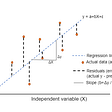## Ridge Regression: Brief Overview## Gradient Descent Math Over Simplified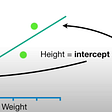## Least Squares: An approach to prediction for Linear Models